# Histogram and boxplot

Institute for Environmental and Spatial Analysis...University of North Georgia

## 1   What is a histogram?

Have you ever heard of a histogram or seen something like this?Statistically speaking, a histogram shows the distribution of data. There are data ranges or bins on the x-axis and count or frequency on the y-axis.

## 2   Bins

Bins in the histogram have an equal width or interval of data. They can be either numeric intervals or categorical classes.

## 3   Frequency

Frequency represents in each bin how often events occur or how many data points are found.

## 4   Drawing a histogram manually

1. Sort data in an ascending order.
3. Count how many data points fall in each bin.
4. Draw a bar at each bin with the bin width as its width and its count as the height.

## 5   Exercise: Manual histogram

Original data

NAME10PopDens
Lanier50
Bryan66
Appling36
Rabun43
Bleckley60
Fayette535
Jefferson32
Seminole34
Camden65
Glynn136
Polk133
Morgan50
Talbot17
Schley30
Union65

Sorted by PopDens

NAME10PopDens
Talbot17
Schley30
Jefferson32
Seminole34
Appling36
Rabun43
Morgan50
Lanier50
Bleckley60
Camden65
Union65
Bryan66
Polk133
Glynn136
Fayette535

Counts for a bin width of 50

ClassPopDensCount
Low[0, 50]8
Medium(50, 100]4
High(100, 150]2
Too High(150, 550]1

## 6   Exercise: Histograms in Excel

There are three methods for creating histograms in Excel:

1. Insert a histogram
2. =FREQUENCY()

### 6.1   Clean up data

2. Open it in Excel and enable editing.3. Delete unnecessary columns.
1. Select column headers A–D and right click ⇒ Delete.
2. Repeat until only NAME10, totpop10, and Sq_Miles columns are left.
4. Select all three columns and adjust their widths by double-clicking the border between any two column headers (red line).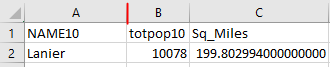5. Decrease decimal in the Sq_Miles column and adjust its width.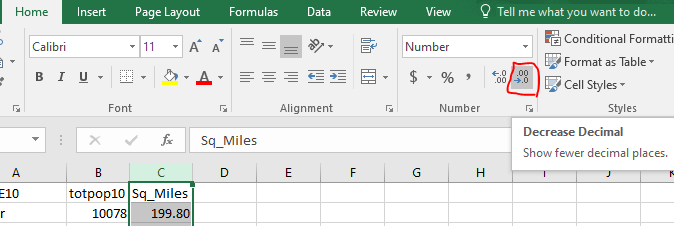### 6.2   Calculate the population density

1. Enter “PopDens10” in cell D1.
2. Enter this formula in cell D2: =B2/C2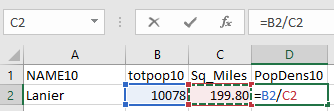3. Copy cell D2 to cells D3 through D160. You can use the little green box in cell D2. Either double-click it or drag it to cell D160.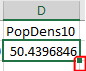4. Decrease decimal in the PopDens10 column.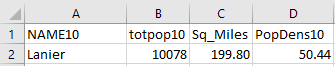### 6.3   “Insert a histogram” method

1. Select the PopDens10 column.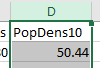2. Insert ⇒ Charts ⇒ Histogram.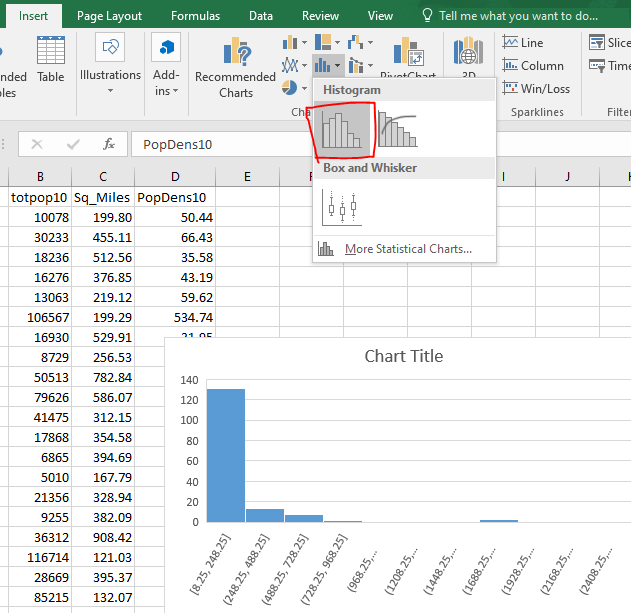3. You can change the bin width.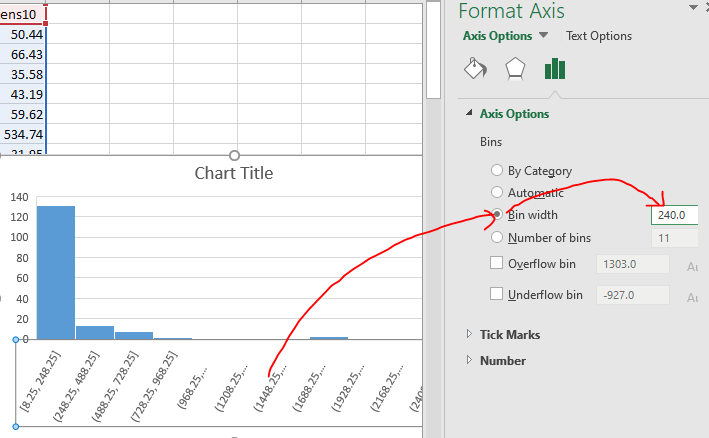### 6.4   “=FREQUENCY()” method

1. For this method, you need to know the minimum and maximum population densities first.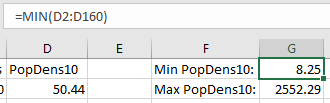1. Enter “Min PopDens10:” and “Max PopDens10:” in cells F1 and F2, respectively.
2. Enter =MIN(D2:D160) and =MAX(D2:D160) in cells G1 and G2, respectively.
2. Enter bins with an interval of 200 starting from 0 up to 2600 in cells G5–G18.
3. Enter labels.
4. Select cells H5–H19. Yes, select one extra cell past the last bin.
5. Enter =FREQUENCY(D2:D160, G5:G18) and hit Ctrl+Shift+Enter.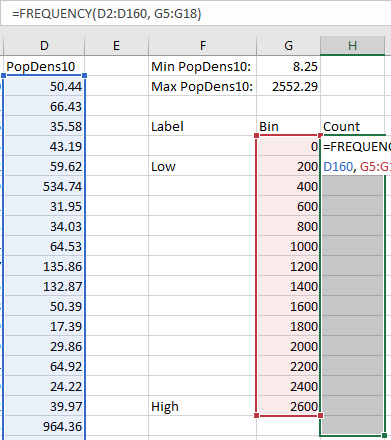6. Select cells F6–F18 and H6–H18. Use Ctrl to select disconnected cell groups.
7. Insert ⇒ Charts ⇒ 2-D Column.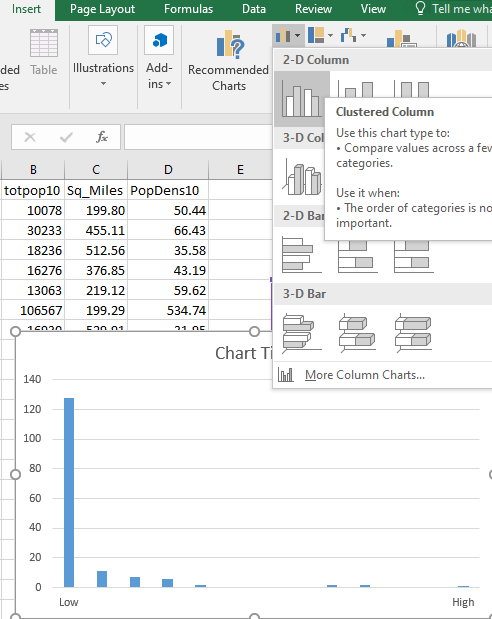### 6.5   “Data analysis add-in” method

1. File ⇒ Options ⇒ Add-ins ⇒ Go... ⇒ Check “Analysis ToolPak” ⇒ OK.
2. Data ⇒ Analysis ⇒ Data Analysis.
3. Histogram ⇒ OK.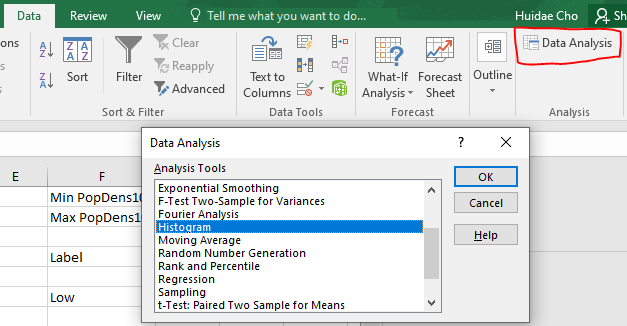4. Input Range: D2:D160
5. Bin Range: G5:G18
6. Check “Chart Output”.
7. OK.
8. A new worksheet will be created with the histogram of cells D2–D160.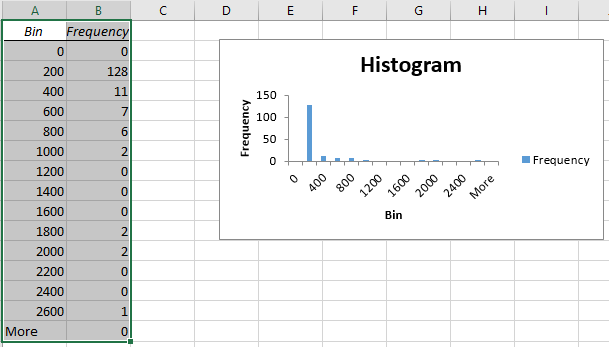## 7   Drawing a boxplot manually

1. Sort data in an ascending order.
2. Add two new columns: Count and Percentile
3. For each row, count the number of data points above and in the current row.
4. Enter the count in the Count column.
5. Divide the count by the total number of rows and multiply it by 100.
6. Enter the percentile in the Percentile column.
7. Find the middle row if there are an odd number of records. Otherwise, calculate the average of two middle rows. This is the median.
8. Repeat the median calculation for the upper and lower halves. They are the 1st (Q1) and 3rd (Q3) quartiles, respectively.
9. Calculate the inter-quartile range by subtracting Q3 from Q1. $\text{IQR}=\text{Q3}-\text{Q1}$
10. Identify outliers falling outside 1.5IQR from Q1 and Q3.
11. Draw a box using Q1 and Q3.
12. Draw whiskers using the lower and upper extremes.
13. Draw outliers if any.

There are different methods for calculating quartiles, but we will use this simple method.

## 8   Exercise: Manual boxplot

Original data

NAME10PopDens
Lanier50
Bryan66
Appling36
Rabun43
Bleckley60
Fayette535
Jefferson32
Seminole34
Camden65
Glynn136
Polk133
Morgan50
Talbot17
Schley30
Union65

Sorted by PopDens and calculated percentiles

NAME10PopDensCountPercentileNote
Talbot1716.7Lower extreme
Schley30213.3
Jefferson32320.0
Seminole34426.7Q1
Appling36533.3
Rabun43640.0
Morgan50853.3
Lanier50853.3Median
Bleckley60960.0
Camden651173.3
Union651173.3
Bryan661280.0Q3, Upper extreme
Polk1331386.7Upper outlier
Glynn1361493.3Upper outlier
Fayette53515100.0Upper outlier
• $\text{IQR} = 66 - 34 = 32$
• Lower outliers: Below $34 - 1.5\times 32 = -14$. There are no lower outliers.
• Upper outliers: Above $66 + 1.5\times 32 = 114$. Polk, Glynn, and Fayette are upper outliers.

## 10   Homework: Histogram and boxplot

1. Create a histogram of Georgia population by county with five labels: Very low, low, medium, high, very high.
1. The minimum and maximum are 8.25 and 2552.29, respectively.
2. Divide 2600 by 5. That is your bin width.
3. Create a new histogram.
2. Create two boxplots for 2010 and 2020 Georgia population by county.
1. Find the most recent Georgia population data by county. Just google “Georgia population by county” and use the first link.
2. Copy and paste this data into a new Excel worksheet.
3. Sort by county.
4. Copy the 2020 population column into the 2010 population worksheet.
5. Calculate the 2020 population density.
6. Use the Excel boxplot tool to create two boxplots side by side.

Please submit two separate Excel files:

1. Histogram.xlsx
2. Boxplots.xlsx